Next Article in Journal
A Decentralized Heuristic Approach towards Resource Allocation in Femtocell Networks
Previous Article in Journal
Spatially-Explicit Bayesian Information Entropy Metrics for Calibrating Landscape Transformation Models

Entropy 2013, 15(7), 2510-2523; https://doi.org/10.3390/e15072510

Article
Improved Minimum Entropy Filtering for Continuous Nonlinear Non-Gaussian Systems Using a Generalized Density Evolution Equation
byMifeng Ren 1,Jianhua Zhang 2,*,Fang Fang 1,Guolian Hou 1 andJinliang Xu 3
1
School of Control and Computer Engineering, North China Electric Power University, Beijing 102206, China
2
State Key Laboratory of Alternate Electrical Power System with Renewable Energy Sources, North China Electric Power University, Beijing 102206, China
3
The Beijing Key Laboratory of New and Renewable Energy, North China Electric Power University, Beijing 102206, China
*
Author to whom correspondence should be addressed.
Received: 26 April 2013; in revised form: 31 May 2013 / Accepted: 19 June 2013 / Published: 25 June 2013

## Abstract

:
This paper investigates the filtering problem for multivariate continuous nonlinear non-Gaussian systems based on an improved minimum error entropy (MEE) criterion. The system is described by a set of nonlinear continuous equations with non-Gaussian system noises and measurement noises. The recently developed generalized density evolution equation is utilized to formulate the joint probability density function (PDF) of the estimation errors. Combining the entropy of the estimation error with the mean squared error, a novel performance index is constructed to ensure the estimation error not only has small uncertainty but also approaches to zero. According to the conjugate gradient method, the optimal filter gain matrix is then obtained by minimizing the improved minimum error entropy criterion. In addition, the condition is proposed to guarantee that the estimation error dynamics is exponentially bounded in the mean square sense. Finally, the comparative simulation results are presented to show that the proposed MEE filter is superior to nonlinear unscented Kalman filter (UKF).
Keywords:
non-Gaussian systems; stochastic filtering; generalized density evolution equation; exponentially mean-square boundedness

## 1. Introduction

State estimation theory has been regarded as an important research area in modern control systems. In particularly, the appearance of the Kalman filtering theory in the last century had a profound influence on modern optimal control [1,2,3]. Kalman filter algorithm is based on the minimum variance estimation for the linear Gaussian systems. However, the noises in real systems may be non-Gaussian; and even for a nonlinear system with Gaussian noises, the system output could be a non-Gaussian variable. In these cases, Kalman filter may lead to poor estimation.
Some attempts have been made at studying filtering algorithms for nonlinear systems with non-Gaussian noises. The existed methodologies to design filters for nonlinear non-Gaussian systems can be classified into three kinds: analytical approach, simulation-based approach and adaptive approach.
Analytical approaches to filtering for nonlinear non-Gaussian systems were investigated in [4,5,6,7]. In [4,5], nonlinear non-Gaussian systems were described by combining an improved square-root B-spline model with a further nonlinear dynamic model. Once B-spline expansions have been made for probability density functions (PDFs), further modeling was still needed to reveal the relationship between the input and the weights related to the PDFs, a nonlinear filter was then constructed by minimizing the error between the measured output PDF and estimated output PDF. Although the proposed filter is suitable to nonlinear non-Gaussian systems, the output PDF should be measureable. Moreover, it is not easy to build the state space expression of the weights related to the PDFs in practical systems. The filter for a class of multivariate dynamic stochastic systems with non-Gaussian stochastic input and nonlinear output was investigated in  and  respectively. In , a new formulation of the residual PDF was made to link the residual PDF to the gain matrix of the filter, and the optimal filtering gain matrix was then solved by minimizing the entropy of the residual. The minimum entropy filter in  presented a good performance in reducing the randomness of the filter residual, and was more general and suited for non-Gaussian systems. However, minimum entropy criterion may not guarantee that the estimation errors approach to zero. In , following the minimum information divergence criterion, a hybrid characteristic function of the conditional estimation error was introduced to construct the performance index of the tracking filter. An analytical solution of the filter gain matrix was then obtained so that the PDFs of the filtering error can follow a target distribution shape. Nevertheless, it is a little complicated to calculate the analytical solution.
There are two types of simulation-based approaches to filtering for nonlinear non-Gaussian systems: numerical integration and sequential Monte Carlo. Although the filtering problem can be tackled using numerical integration [8,9], it is difficult to implement when the dimension of the state vector is higher. Sequential Monte Carlo simulation [10,11,12,13,14,15], which is also named particle filtering strategy, has shown its great advantages to deal with filtering problems for nonlinear non-Gaussian systems. Nevertheless, there are still many issues to be solved, for example, (1) random sampling may bring the accumulation of Monte Carlo error and even lead to filter divergence; (2) a large number of particles are needed to avoid degradation and to improve the estimation accuracy, which makes the calculation a sharp increase.
Adaptive approaches have been also investigated for solving filtering problem in nonlinear non-Gaussian systems in last decades. Since minimum error entropy criterion ensures that the estimation error has small uncertainty, it was used for supervised training of nonlinear stochastic system in [16,17]. However, entropy does not change with the mean of the distribution, the algorithm may not yield zero-mean error. Therefore, the result may be corrected by properly modifying the bias of the output processing element of the neural networks. Maximum mutual information criterion was proposed for adaptive filtering in , this criterion is robust to measure distortions. Nevertheless, the maximum mutual information criterion leads to non-unique optimum solution. It is necessary to use a priori information about the unknown system in order to obtain unique solution.
In general, the filtering problem for nonlinear non-Gaussian systems calls for further investigation. The filtering problem addressed here is solved by combining the improved performance index with optimal design method.
Entropy is a natural extension beyond mean square error because it is a function of PDF. One of the most important problems for minimum entropy filtering is the formulation of the PDF of estimation error. For continuous nonlinear systems, the classical Liouville equation and its Dostupov-Pugachev extension have been used to obtain the PDF of the concerned stochastic variable. However, it is very difficult to have their analytical/numerical solutions due to high-dimensional partial differential equations. From the viewpoint of the random event description of the principle of preservation of probability, the generalized density evolution equation was developed in . Different from the traditional density evolution equations, the dimensions of the generalized density evolution equation just depend on the necessary physical quantities' dimensions not the whole original systems itself, which makes the dimensions of the partial differential equations much lower. Some application results (see e.g., [20,21]) illustrated the efficiency and conveniences of the formulated density evolution equation.
The contribution of this paper is to develop a new filtering strategy for multivariable nonlinear systems with non-Gaussian disturbances by utilizing a novel performance index which contains the entropy of estimation error, square error and constraints on gain matrix of the filter. A novel approach, which uses the principle of preservation of probability, is presented here to formulate the joint PDF evolution equation of the estimation errors. The PDF evolution equation explicitly reveals the relationship among the estimation errors of the filter, filter gain and random inputs. In addition, the entropy of estimation errors in the performance index is replaced by its information potential presented in [16,17] so as to simplify the calculation of the entropy. The filter gain matrix for nonlinear non-Gaussian systems is then designed by minimizing the proposed performance index. This filtering algorithm yields to the estimation errors which not only have small uncertainty but also approach to zero. Finally, the exponentially boundedness in the mean square sense is analyzed for the estimation error dynamics.
This paper is organized as follows: in section 2, the state-space model of a nonlinear non-Gaussian system and the structure of filter are built to formulate the filtering problem. The joint PDF evolution of estimation errors is formulated in Section 3 so as to calculate the entropy of estimation errors. The filtering algorithm is proposed in Section 4 by minimizing the improved entropy criterion and the exponentially mean-square boundedness condition for error systems is provided. Comparative simulation results are given to illustrate the efficiency and validity of the proposed method in Section 5. Conclusions are drawn in Section 6. Notation. ℜn and ℜn×m denote the n-dimensional Euclidean space and the set of all n × m real matrices respectively. The superscript “T” denotes the transpose. If A is a matrix, λmax (·) and λmin (·) represent the largest and smallest eigenvalue of A respectively. E(·) stands for the mathematical expectation of random variables.

## 2. Problem Formulation

#### 2.1. System Model

Consider a nonlinear stochastic continuous system described by:
${ x ˙ ( t ) = A ( t ) x ( t ) + D ( t ) ω ( t ) y ( t ) = F ( x ( t ) , υ ( t ) )$
where x ∈ ℜn is the state vector, y ∈ ℜm is the measured output vector. ω ∈ ℜp and υ ∈ ℜq are system noises and measurement noises respectively. A(t) and D(t) are two known time-varying system matrices. The following assumptions are made for simplicity, which can be satisfied by many practical systems.
Assumption 1: F(·) is a known Borel measurable and smooth vector-value nonlinear function of its arguments.
Assumption 2: ω and υ are bounded, mutually independent random vectors with known joint PDFs γω and γυ.

#### 2.2. Filter Dynamics

For the nonlinear stochastic system (1), the filter dynamics can be formulated as follows:
${ x ^ ˙ ( t ) = A ( t ) x ^ ( t ) + L ( y ( t ) − y ^ ( t ) ) y ^ ( t ) = F ( x ^ ( t ) , 0 )$
The resulting estimation errorsatisfies: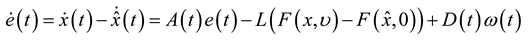where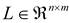is the gain matrix to be determined and can be denoted as, Li is the ith row vector of L. Let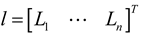and thusis a stretched column vector.
The purpose of filtering algorithm for nonlinear non-Gaussian systems is to ensure that the estimation errors achieve small dispersion and approach to zero. Entropy provides a measure of the average information contained in a given PDF. When entropy of estimation errors is minimized, all moments of the error PDF are constrained. Hence, the following quadratic Renyi’s entropy of estimation error is still contained in the performance index to measure the dispersion of the estimation error:where Pe(z) is the joint PDF of estimation error and V(e) the information potential . It can be seen that it is necessary to provide the joint PDF of errors firstly. In the next section, we will deduce the expression of Pe(z) according to the generalized density evolution equation.

## 3. Formulation for the Joint PDF of Error

In most cases, Equation (3) is a well-posed equation, and the error vector e(t) can be determined uniquely. It may be assumed to be:At the present stage, the explicit expression of H(·) is not requisite, and the sufficient condition just needs to know its existence and uniqueness. The derivative of e(t) can be assumed to take the form:It is observed that all the randomness involved in this error dynamics comes from noises ω and υ, thus, the augmented system (e(t), ω, υ) is probability preserved. In other words, from the random event description of the principle of preservation of probability, it leads to:where Ωe, Ωω and Ωυ are the distribution domains of e, ω and υ, respectively;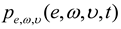is the joint PDF of (e(t), ω, υ). It follows from Equation (7) that:
$D D t ∫ Ω e × Ω ω × Ω υ p e ω υ ( e , ω , υ , t ) d e d ω d υ = ∫ Ω e × Ω ω × Ω υ ( ∂ p e ω υ ∂ t + h ∂ p e ω υ ∂ e ) d e d ω d υ$
Combining Equation (7) with Equation (8) and considering the arbitrariness of, it yields:where Equation (6) and Equation (9) are the generalized density evolution equation (GDEE) for (e(t), ω, υ). The corresponding instantaneous PDF of (e(t)) can be obtained by solving a family of partial differential equations with the following given initial condition:where δ(·) is the Dirac-Delta function; andis deterministic initial value of (e(t)). Then, we have:where the joint PDFis the solution of (8), which can be obtained according to the method presented in .

## 4. Improved MEE Filtering

#### 4.1. Performance Index

The following performance index for a minimum entropy filter was presented in :where R1 and R2 are weighing matrices. The first term is the entropy of the estimation errors and the second term is used to penalize the elements of the gain. Although the MEE criteria can minimize both the probabilistic uncertainty and dispersion of the estimation error, it cannot guarantee the minimum error. In this work , an improved performance index J is considered:whereis the quadratic Renyi’s entropy given in Equation (4).is the weight corresponding to the mean squared error. The third term on the right side of the equation is utilized to make the estimation errors approach to zero. Since Renyi’s entropy is a monotonic increasing function of the negative information potential, minimization of Renyi’s entropy is equivalent to minimizing the inverse of the quadratic information potential, so the performance index can be rewritten as follows:Remark 1: The entropy of estimation error is replaced by quadratic information potential of error in order to simplify the calculation. Nonparametric estimation of the PDF of estimation errors is another alternative way to estimate the PDF except the method introduced in . Nonparametric entropy (or information potential) estimation of random error can be found in [16,17].

#### 4.2. Optimal Filter Gain Matrix

After constructing the performance index J, the optimal filter gain can be carried out by minimizing the performance index Equation (14), which can be regarded as an unconstrained nonlinear programming problem. The classical conjugate gradient approach is employed here to obtain an iterative solution, which could avoid the difficulty of solving the extremum condition (). The elements of optimal filter gain can be solved and summarized in Theorem 1.
Theorem 1: For a given accuracy Ɛ > 0, the gain matrix of the suboptimal improved MEE filter is given by:where,and the Hessian matrixis: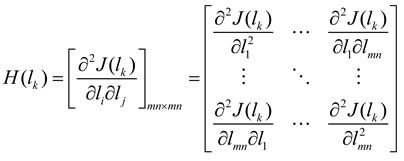Proof: Choose an initial value l0, and denote k := 0. Search the next value lk+1 from the given point lk along the conjugate gradient direction Pk. Repeat the above procedure until reach the given accuracy Ɛ. Consequently, we can obtain the optimal filtering algorithm shown in Equation (15).
Based on the conjugate gradient method, the steps to solve the optimal filter gain are summarized as follows:
Step (1) Give an accuracy Ɛ > 0 and initialize l0, denote k = 0
Step (2) Set.
Step (3) Obtainby solving, and update the gain.
Step (4) If, stop, and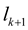is the optimal solution; Otherwise, if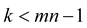, turn to step (5), and if, reset, and turn to step (2).
Step (5) Choose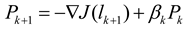, set, and turn to step 3), where.
Remark 2: The conjugate gradient method here could overcome the slow convergence of the steepest descent approach. It is one of the most effective algorithms to deal with large-scale nonlinear optimization problems.

#### 4.3. Exponentially Bounded in the Mean Square

In this section, the Lyapunov method is utilized to give the condition under which the filter error dynamics is exponentially ultimately bounded in the mean square. Firstly, in order to simplify the problem, we give the following assumption:
Assumption 3: The nonlinear vector functionisassumed to satisfyand:where,are known constant matrices,,are vectors, a is known positive constant.
For estimation error dynamics (3), the exponentially mean-square boundedness is defined as follows:
Definition 1 : For all initial conditions, the dynamics of the estimation error (i.e., the solution of the system (3)) is exponentially ultimately bounded in the mean square if there exist constants α > 0, β > 0 and γ > 0 such that:Moreover, filter (2) is said to be exponential if, for every, system (3) is exponentially ultimately bounded in the mean square.
Theorem 2: Let the filter parameter L be given. If there exist positive scalars Ɛ1, Ɛ2 and Ɛ3 such that the following matrix inequality:has a positive definite solution P > 0, then system (3) is exponentially ultimately bounded in the mean square.
Proof: Fixarbitrarily and denote. The Lyapunov function candidate can be chosen as:where P > 0 is the solution to the matrix inequality Equation (19).
For notational convenience, we give the following definitions:and then it follows from Equation (3) that:Hence, the derivative of V along a given trajectory Equation (22) is obtained as:Let Ɛ1, Ɛ2 and Ɛ3 be positive scalars. Then the following matrix inequalities hold:Noticing Assumptions 3 and Equation (17), we have: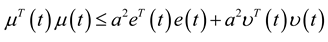Substituting Equations (24)–(27) into Equation (23) results in:where:and it is known from Equation (19) that II < 0.
Based on Equation (28), it can be found that:Let. Integrating both sides from 0 to T > 0 and taking the expectation lead to:where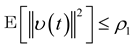,(according to Assumption 2, ω(t) and υ(t) are bounded random vectors) ,and:Notice that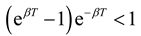and let,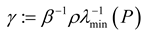. Since T > 0 is arbitrary, the definition of exponential ultimate boundedness in (18) is then satisfied, and this completes the proof of Theorem 2.

## 5. Simulation Results

In order to show the applicability of the proposed filtering algorithm, we consider the following nonlinear system represented as :whereand. The random disturbances ω and υ obey non-Gaussian, and their distributions are shown in Figure 1. The weights in Equation (14) are selected as R1 = 10, R2 = 2 and R3 = 10, respectively. The simulation results based on the MEE filter are shown in Figure 2, Figure 3, Figure 4, Figure 5, Figure 6, Figure 7a and Figure 8a). And the comparative results between MEE filter and UKF are shown in Figure 7 and Figure 8.
Figure 2 demonstrates that the performance index decreases monotonically with time. In Figure 3 and Figure 4, both the range and PDFs of errors are given, it can be seen that the shapes of PDFs of the tracking errors become narrower and sharper along with the increasing time, which illustrates the dispersions of estimation errors can be reduced. In order to clarify the improvements, the initial and final PDFs are shown in Figure 5 and Figure 6. It can be shown from Figure 3, Figure 4, Figure 5 and Figure 6 that the proposed improved MEE filter can decrease the uncertainties of the estimation errors and drive the estimation errors approaching to zero. Figure 7 shows that both the MEE filter and UKF make the estimation errors approach to zero. But the estimation errors in a are more closer to zero with smaller randomness. The variances of the filter gains in two cases are presented in Figure 8. It can be seen that the gains of UKF are not convergent.
From the above analyses, it is obvious that the proposed strategy has better performance than UKF. Therefore, the proposed MEE filter is more suitable for nonlinear stochastic systems with non-Gaussian noises.
Figure 1. Distribution of random noises ω and υ
Figure 1. Distribution of random noises ω and υ
Figure 2. Performance index.
Figure 2. Performance index.
Figure 3. PDF of estimation error e1
Figure 3. PDF of estimation error e1
Figure 4. PDF of estimation error e2.
Figure 4. PDF of estimation error e2.
Figure 5. Initial and final PDFs of e1
Figure 5. Initial and final PDFs of e1
Figure 6. Initial and final PDFs of e2
Figure 6. Initial and final PDFs of e2
Figure 7. Estimation errors under the filter: (a) MEE filter (b) UKF.
Figure 7. Estimation errors under the filter: (a) MEE filter (b) UKF.
Figure 8. Filter gain: (a) MEE filter (b) UKF.
Figure 8. Filter gain: (a) MEE filter (b) UKF.

## 6. Conclusions

In this paper, based on the improved MEE criterion, optimal filter design problem is studied for multivariate nonlinear continuous stochastic systems with non-Gaussian noises. A novel performance index, including Renyi’s entropy of the estimation error, mean value of the squared error and constrains on filter gain, is formulated. By using the generalized density evolution equation, the relationship among the PDFs of estimation error, random disturbances and filter gain matrix is obtained. A recursive optimal filtering algorithm is obtained by minimizing the improved MEE criterion. The exponentially mean-square boundedness condition of the estimation error systems is established. In this work, the proposed MEE filter and UKF are both applied to a continuous nonlinear and non-Gaussian stochastic system. Comparative simulation results demonstrate the superiority of the presented control algorithm.

## Acknowledgments

This work was supported by National Basic Research Program of China under Grant (973 Program 2011CB710706), China National Science Foundation under Grant (60974029), the Natural Science Foundation of China of International Cooperation Project (51210011) and the Fundamental Research Funds for the Central Universities. These are gratefully acknowledged.

## Conflict of Interest

The authors declare no conflict of interest.

## References

1. Kalman, R.E. A new approach to linear filtering and prediction problems. Trans. ASME J. Basic Eng. 1960, 82, 35–45. [Google Scholar] [CrossRef]
2. Kalman, R.E.; Bucy, R.S. New results in linear filtering and prediction theory. Trans. ASME J. Basic Eng. 1961, 83, 95–107. [Google Scholar] [CrossRef]
3. Hoblos, V.G.; Chafouk, H. A gain modification form for Kalman filtering under state inequality constraints. Int. J. Adapt. Control 2010, 24, 1051–1069. [Google Scholar]
4. Guo, L.; Wang, H. Fault detection and diagnosis for general stochastic systems using B-spline expansions and nonlinear filters. IEEE Trans. Circ. Syst. I 2005, 52, 1644–1652. [Google Scholar]
5. Li, T.; Guo, L. Optimal fault-detection filtering for non-gaussian systems via output PDFs. IEEE Trans. Syst. Man Cybern. Syst. Hum. 2009, 39, 476–481. [Google Scholar]
6. Guo, L.; Wang, H. Minimum entropy filtering for multivariate stochastic systems with non-Gaussian noises. IEEE Trans. Automat. Contr. 2006, 51, 695–700. [Google Scholar] [CrossRef]
7. Zhou, J.; Zhou, D.; Wang, H.; Guo, L.; Chai, T. Distribution function tracking filter design using hybrid characteristic functions. Automatica 2010, 46, 101–109. [Google Scholar] [CrossRef]
8. Kitagawa, G. Non-Gaussian state-space modeling of nonstationary time-series. J. Am. Stat. Assoc. 1987, 82, 1032–1063. [Google Scholar]
9. Kramer, S.C.; Sorenson, H.W. Recursive Bayesian estimation using piece-wise constant approximations. Automatica 1988, 24, 789–801. [Google Scholar] [CrossRef]
10. Tanizaki, H.; Roberto, S. Mariano, Nonlinear and Non-Gaussian State-space Modelling with Monte-Carlo Simulations. J. Econometrics 1998, 83, 263–290. [Google Scholar] [CrossRef]
11. Kitagawa, G. Monte carlo filter and smoother for non-Gaussian nonlinear state space models. J. Comput. Graph. Stat. 1996, 5, 1–25. [Google Scholar]
12. Cappe, O.; Godsill, S.; Moulines, E. An overview of existing methods and recent advances in sequential Monte Carlo. Proceedings of the IEEE 2007, 95, 899–924. [Google Scholar] [CrossRef]
13. Vaswani, N. Particle filtering for large dimensional state spaces with multimodal observation likelihoods. IEEE Trans. Signal Proces. 2008, 56, 4583–4597. [Google Scholar] [CrossRef]
14. Tang, X.; Huang, J.; Zhou, J.; Wei, P. A sigma point-based resampling algorithm in particle filter. Int. J. Adapt. Control 2012, 26, 1013–1023. [Google Scholar] [CrossRef]
15. Zhao, S.Y.; Liu, F.; Luan, X.L. Risk-sensitive filtering for nonlinear Markov jump systems on the basis of particle approximation. Int. J. Adapt. Control 2012, 26, 158–170. [Google Scholar] [CrossRef]
16. Erdogmus, D.; Principe, J.C. An error-entropy minimization algorithm for supervised training of nonlinear adaptive systems. IEEE Trans. Signal Proces. 2002, 50, 1780–1786. [Google Scholar] [CrossRef]
17. Principe, J.C. Information Theoretic Learning: Renyi’s Entropy and Kernel Perspectives; John Wiley & Sons: New York, NY, USA, 2010. [Google Scholar]
18. Chen, B.; Hu, J.; Li, H.; Sun, Z. Adaptive filtering under maximum mutual information criterion. Neurocomputing 2008, 71, 3680–3684. [Google Scholar] [CrossRef]
19. Li, J.; Chen, J. The principal of preservation of probability and the generalized density evolution equation. Struct. Saf. 2008, 30, 65–77. [Google Scholar]
20. Peng, Y.; Li, J. Exceedance probability criterion based stochastic optimal polynomial control of Duffing oscillators. Int. J. Nonlin. Mech. 2011, 46, 457–469. [Google Scholar] [CrossRef]
21. Li, J.; Peng, Y.; Chen, J. A physical approach to structural stochastic optimal controls. Probabilist. Eng. Mech. 2010, 25, 127–141. [Google Scholar] [CrossRef]
22. Li, J.; Chen, J. Stochastic Dynamics of Structures; John Wiley & Sons: New York, NY, USA, 2009. [Google Scholar]
23. Wang, Z.; Ho Daniel, W.C. Filtering on nonlinear time-delay stochastic systems. Automatica 2003, 39, 101–109. [Google Scholar] [CrossRef]
24. Mao, X. Stochastic Differential Equations and Applications; Harwood University: New York, NY, USA, 1997. [Google Scholar]